### Linear indpendence of characters

Posted on May 18, 2019

Theorem. Let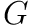be an abelian group, let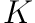be a field, and suppose that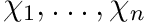are distinct characters ofwith values in, i.e.,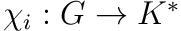is group homomorphism for each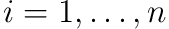. Then the characters are linearly independent, i.e., if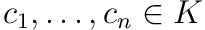such that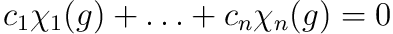for all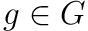, then it must be that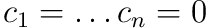.

If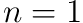observe that since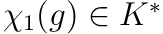in particular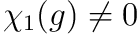for all. Thus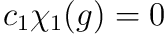implies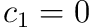.

Suppose that for some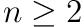all sets of fewer than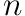characters ofare linearly indpendent. Letbe distinct characters andsuch thatfor all. Let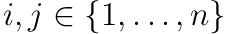be distinct. Observe that since the characters are distinct there exists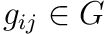such that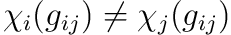. Observe that by our choice of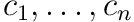we haveandfor all. Therefore subtracting the two we see thatfor all. Since the term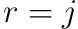vanishes, by the inductive hypothesis we must have all the coefficients of the linear combination equal to zero. That is, we must have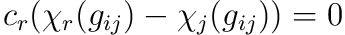for all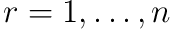. In particular,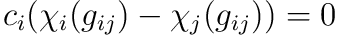. But by our choice of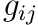we have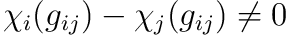. Thus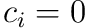.

The above proof is inspired by the proof given in (1, Theorem 2.1).